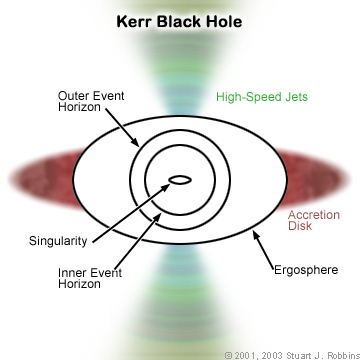The term singularity in science is defined as the point in space where some properties of the said point in space is infinite. There are many occurrences in space that has the capacity of singularity. One classic example of this concept is the black hole. A black hole is the result of a dead star that has used up all of its energy and has died already.

Because it needs energy, it then sucks different elements near the hole to gain size and power. One thing to note about this is the center of the black hole, which, by virtue of the Classical Theory of Astronomy, states that a finite mass of a certain celestial object when compressed becomes a mass of zero and also results in an infinite density.

Another example is that of the Big Bang Theory. When the properties are observed and inferred from what was gained based on the theory, the results of both the mass and density of the universe caused by the occurrence results to infinity.

The concept of singularity is part of a classical theory. Though as of late, there is yet a theory that can properly explain this concept. Also, it is also said that the possibility of avoiding an occurrence such as this can be applied through the method of quantum gravity.